Calculations

First, I measured the force exerted on the propeller by the twisted rubber band.  I did this by using a digital scale.

After twisting the rubber band, I placed the outside edge of the propeller on the scale, as shown in the following picture.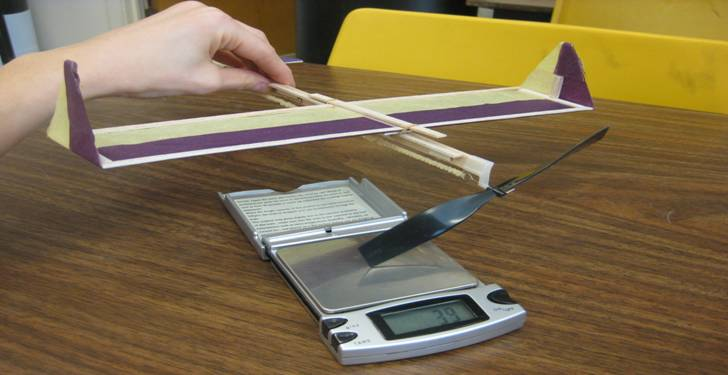The scale measured in grams, so I needed to convert this to Newton’s.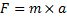Where,

m = the reading on the scale

a = 9.8 m/s2

Then I measured the radius of the propeller and calculated the work.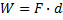Where,

F = the previous calculation

d = 2πr X (number of turns)

Then the power could be calculated by measuring the time it takes for the propeller to unwind.Where,

W = work

t = time in seconds

In order to calculate the transport cost, the glide slope was determined by measuring how far the airplane “glided” when launched without the propeller powering it.  This was done several times to get an average estimate.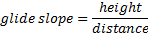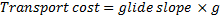Where g is the acceleration due to gravity.

Note: the units of transport cost, m/s2 are equivalent to J/kg/m

Then convert the units to kWh/tonne/km and compare to a Boeing 747.

1kWh = 3.6MJ